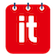# Searching for Using shortcut keys to speed up productivity.

Be more efficient and save a lot of time. Using shortcuts makes you more efficient at doing certain tasks because you're not unnecessarily reaching for a mouse all the time. Once you learn some key combinations, you'll definitely notice a boost to productivity.

Press ? whilst in your schedule to see more.

#### Changing the Schedule View

v then t = Timeline View
v then m = Month View
v then w = Week View
v then d = Day View
v then l = List View

g then t = Go to Today
g then n = Go to Next Dates
g then p = Go to Previous Dates
g then month = Go to month number (01 to 12)
g then year = Go to year (2010 to 2050)

#### Actions

n then e = Add a New Event
n then f = Open Filter
/ = Focus Quick Search Box
? = Show Shortcut Keys Help
Ctrl + S = Save Event

#### Navigating the Timeline View

v then 1 then d = View 1 Day
v then 5 then d = View 5 Days
v then 1 then w = View 1 week
v then 2 then w = View 2 week
v then 3 then w = View 3 week
v then 1 then m = View 1 Month
v then 2 then m = View 2 Months
v then 3 then m = View 3 Months
v then m = Month View
v then w = Week View
v then d = Day View
v then l = List View

Last updated on May 25, 2018Schedule it Ltd/faq/10844/using-shortcut-keys-to-speed-up-productivity